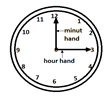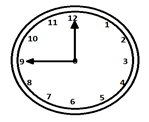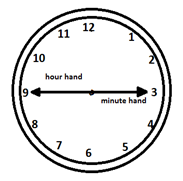# Clock - Solved Examples

Q 1 − The smaller or slower hand of a clock is called hour hand it makes how many times revolution in a day?Options :

A - One

B - Three

C - Four

D - Two

Explanation

There are 24 hrs in a day so each 12 hrs one revolution, so in 24 hrs there will be two revolutions.

Q 2 − The bigger hand of a clock makes how many revolutions every hour?Options :

A - One

B - Two

C - Three

D - Four

Explanation

In every one hour, the bigger hand revolves only once.

Q 3 − How many revolutions that second hand makes per minute?

Options :

A - One

B - Two

C - Three

D - None of these

Explanation

Second hand also revolves once per one minute.

Q 4 − In one hour how much minute space covers the hour hand?

Options :

A - 60

B - 55

C - 45

D - 50

Explanation

60-5 = 55

Q 5 − If the two hands are in opposite direction then what is the difference of degree and min between the two hands?

Options :

A - 180o and 30 min.

B - 180o and 30 min.

C - 60o and 15 min.

D - 120o and 10 minExplanation

When hour hand and minute hand are in a straight line they are 30 min space apart.

Q 6 − What is the value of the angle covered in 60 min?

Options :

A - 360o

B - 300o

C - 180o

D - 90o

Explanation

In general, the angle covered by 1 min = 6o, so the angle covered by 60 minute = 360o

Q 7 − In between 11 and 1 O`clock, how many times both the hands will coincide with each other?

Options :

A - One

B - Two

C - Three

D - None of these

Explanation

Because both hands will coincide each other at 12 O`clock.

Q 8 − How many seconds are acquired when both the hands are 90o space apart?

Options :

A - 870 sec.

B - 880 sec.

C - 890 sec.

D - 900 sec.

Explanation

15 × 60 = 900 sec.

Q 9 − How many hours are there in a year?

Options :

A - 8760 hrs

B - 8765 hrs

C - 8755 hrs

D - 8750 hrs

Explanation

24 × 365 = 8760 hrs.

Q 10 − How many minutes in 365 days?

Options :

A - 525600 min.

B - 525550 min.

C - 52500 min.

D - 5254450 min

Explanation

1 day = 24 hrs. = 1440 min.

365 day = 1440 × 365 = 525600 min.

reasoning_clock.htm